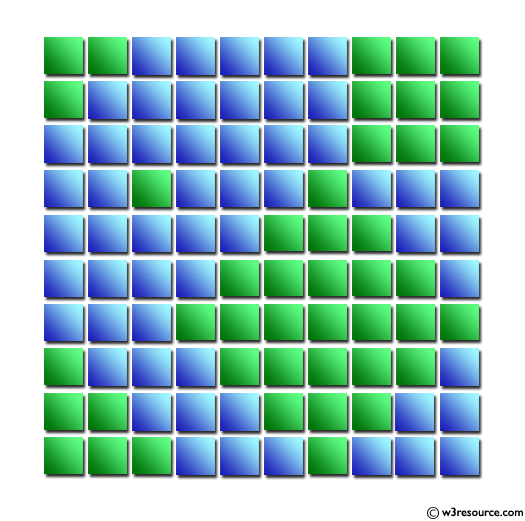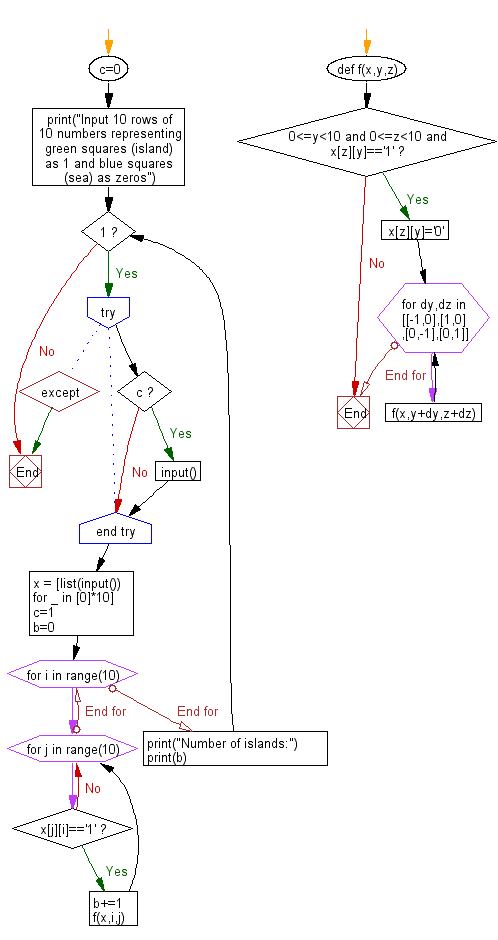﻿ Python: Read the mass data and find the number of islands - w3resource# Python: Read the mass data and find the number of islands

## Python Basic - 1: Exercise-57 with Solution

There are 10 vertical and horizontal squares on a plane. Each square is painted blue and green. Blue represents the sea, and green represents the land. When two green squares are in contact with the top and bottom, or right and left, they are said to be ground. The area created by only one green square is called "island". For example, there are five islands in the figure below.
Write a Python program to read the mass data and find the number of islands.

Input:
A single data set is represented by 10 rows of 10 numbers representing green squares as 1 and blue squares as zeros.
1100000111
1000000111
0000000111
0010001000
0000011100
0000111110
0001111111
1000111110
1100011100
1110001000
Number of islands:
5

Pictorial Presentation:Sample Solution:

Python Code:

``````c=0
def f(x,y,z):
if 0<=y<10 and 0<=z<10 and x[z][y]=='1':
x[z][y]='0'
for dy,dz in [[-1,0],[1,0],[0,-1],[0,1]]:f(x,y+dy,z+dz)
print("Input 10 rows of 10 numbers representing green squares (island) as 1 and blue squares (sea) as zeros")
while 1:
try:
if c:input()
except:break
x = [list(input()) for _ in *10]
c=1;b=0
for i in range(10):
for j in range(10):
if x[j][i]=='1':
b+=1;f(x,i,j)
print("Number of islands:")
print(b)
``````

Sample Output:

```Input 10 rows of 10 numbers representing green squares (island) as 1 and blue squares (sea) as zeros
1100000111
1000000111
0000000111
0010001000
0000011100
0000111110
0001111111
1000111110
1100011100
1110001000
Number of islands:
5
```

Flowchart:Python Code Editor:

Have another way to solve this solution? Contribute your code (and comments) through Disqus.

What is the difficulty level of this exercise?

Test your Programming skills with w3resource's quiz.

﻿

## Python: Tips of the Day

Iterating over dictionaries using 'for' loops:

I am a bit puzzled by the following code: d = {'x': 1, 'y': 2, 'z': 3} for key in d: print key, 'corresponds to', d[key] What I don't understand is the key portion. How does Python recognize ...

key is just a variable name.

```for key in d:
```

For Python 3.x:

```for key, value in d.items():
```

For Python 2.x:

```for key, value in d.iteritems():
```

To test for yourself, change the word key to poop.

In Python 3.x, iteritems() was replaced with simply items(), which returns a set-like view backed by the dict, like iteritems() but even better. This is also available in 2.7 as viewitems().

The operation items() will work for both 2 and 3, but in 2 it will return a list of the dictionary's (key, value) pairs, which will not reflect changes to the dict that happen after the items() call. If you want the 2.x behavior in 3.x, you can call list(d.items()).

Ref: https://bit.ly/37dm0Qo